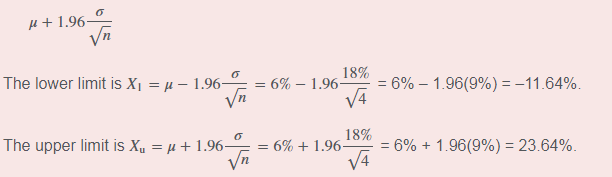# Confidence Interval and Z score

Hi guys,
I’ve ran into a few problems that use different formulas to compute the Confidence interval and the Z score.

Ex: Assume that the equity risk premium is normally distributed with a population mean of 6% and a population std. deviation of 18%. Over the last four years, equity returns (relative to the risk free rate) have averaged -2.0%. You have a large client who is very upset and claims that results this poor should never occur. Evaluate your client’s concerns.
A. Construct a 95% confidence interval around the population mean for a sample of four year returns.
B. What is the probability of a -2.0 percent or lower average return over a four year period?

For the question A, they use the below formulaHowever, I dont understand why they use the population mean to compute the confidence interval. Don’t we use the point estimate (sample mean)?

For the question B,

The answer used the equation “z = (observation - population mean)/(Std error)”
where std error = (std deviation/Sqrt(population size)) to compute the z score while I used the first equation [(z = observation - population mean)/ std deviation]

How would I know which one to use? they gave significantly different answers.

I’m very grateful for your help!!